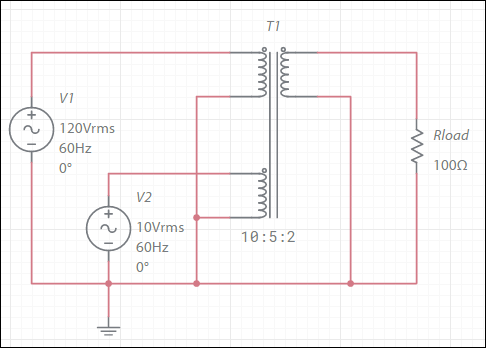# Singular matrix

A singular matrix is a condition that arises when the system of mathematical equations describing the circuit has either no solution or an infinite number of solutions. This can occur as a result of round-off errors as the simulator attempts to solve the circuit, but more commonly it is a result of problems with the circuit configuration. Configurations that can lead to singular matrix errors include:

• Parallel voltage sources
• Series current sources
• Parallel inductors during DC operating point (as inductors are treated as short circuits)
• Floating nodes (those with no DC path to ground)

In general, Multisim Live detects these conditions prior to starting the simulator, and shows appropriate warnings and errors.

If you encounter a singular matrix error message, ensure that the RSHUNT setting is enabled, otherwise you might introduce floating nodes into the simulation.

Also ensure that your connections are mathematically valid. For example, consider the following circuit:Since the voltage at the primary-side lower coil is fixed at 5/10 of the voltage at the primary-side upper coil, both cannot be driven by voltage sources. This is invalid and is equivalent to having voltage sources in parallel.